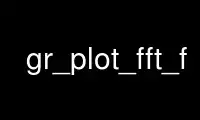# gr_plot_fft_f - Online in the CloudThis is the command gr_plot_fft_f that can be run in the OnWorks free hosting provider using one of our multiple free online workstations such as Ubuntu Online, Fedora Online, Windows online emulator or MAC OS online emulator

### PROGRAM:

#### NAME

gr_plot_fft_f - plot complex binary data using GNU Radio

#### SYNOPSIS

gr_plot_fft_f: [options] input_filename

#### DESCRIPTION

Takes a GNU Radio complex binary file and displays the I&Q data versus time as well as the
frequency domain (FFT) plot. The y-axis values are plotted assuming volts as the amplitude
of the I&Q streams and converted into dBm in the frequency domain (the 1/N power
adjustment out of the FFT is performed internally). The script plots a certain block of
data at a time, specified on the command line as -B or --block. This value defaults to
1000. The start position in the file can be set by specifying -s or --start and defaults
to 0 (the start of the file). By default, the system assumes a sample rate of 1, so in
time, each sample is plotted versus the sample number. To set a true time and frequency
axis, set the sample rate (-R or --sample-rate) to the sample rate used when capturing the
samples.

#### OPTIONS

-h, --help
show this help message and exit

-B BLOCK, --block=BLOCK
Specify the block size [default=1000]

-s START, --start=START
Specify where to start in the file [default=0]

-R SAMPLE_RATE, --sample-rate=SAMPLE_RATE
Set the sampler rate of the data [default=1.0]

Use gr_plot_fft_f online using onworks.net services

Free Servers & Workstations